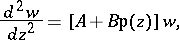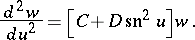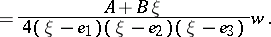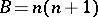# Lamé equation

A linear ordinary second-order differential equation in the complex domain(1)

whereis the Weierstrass-function andandare constants. This equation was first studied by G. Lamé ; it arises in the separation of variables for the Laplace equation in elliptic coordinates. Equation (1) is also called the Weierstrass form of the Lamé equation. By a change of the independent variable in (1) one obtains Jacobi's form of the Lamé equation:There are also numerous algebraic forms of the Lamé equation, transition to which is carried out by various transformations of the independent variable in (1), for example:(2)For practical applications the Jacobi form is the most suitable.

Especially important is the case when in (1) (or (2)), whereis a natural number. In this case the solutions of (1) are meromorphic in the whole plane and their properties have been thoroughly studied. Among the solutions of (2) withthe Lamé functions are of paramount importance (cf. Lamé function).